Chapter 8 Class 6 Decimals
Concept wise

Decimal are number which have a decimal point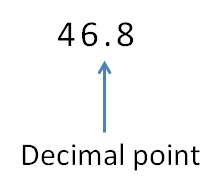Decimal number are number between two whole numbers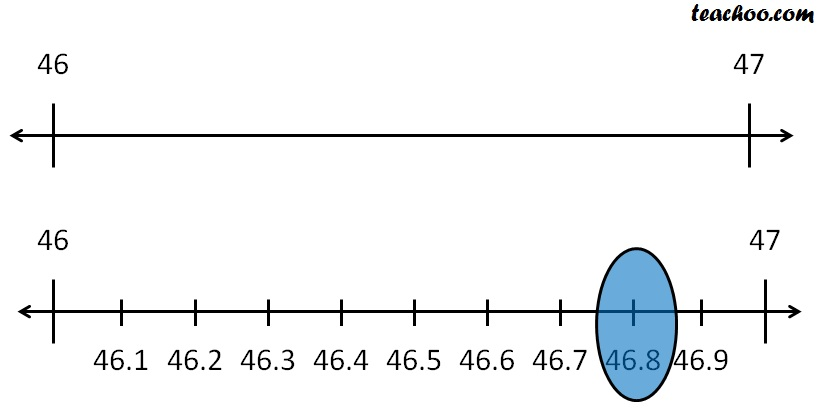But, what does decimal point mean ?

Let ‘s look at place value first

#### Place value of numbers with decimal point

For number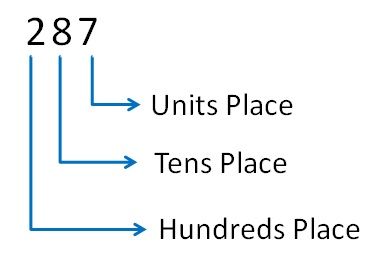The numbers  is

Two hundred eighty seven

287 = 2 × 100 + 8 × 10  + 7

So,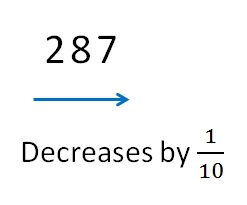Suppose we put a decimal point as well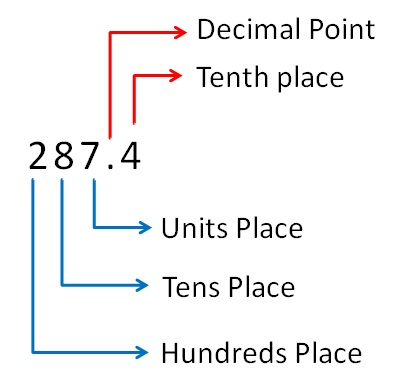The numbers  is

Two hundred eighty seven point four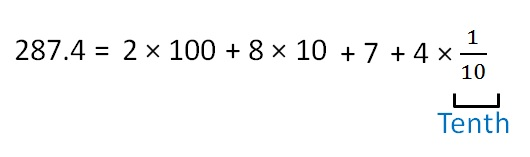Similarly,

We can have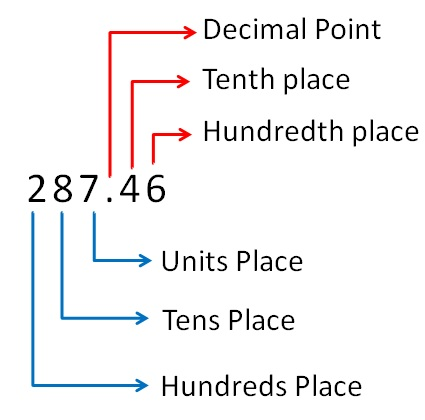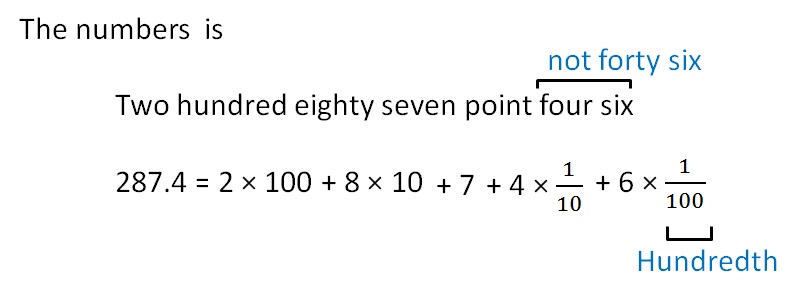Learn in your speed, with individual attention - Teachoo Maths 1-on-1 Class

### Transcript

Similarly, We can have The numbers is Two hundred eighty seven point four six 287.4 = Decimal Point Tenth place Hundredth place